# 最小二乘法线性回归：矩阵视角¶

## 极大似然估计和最小二乘法¶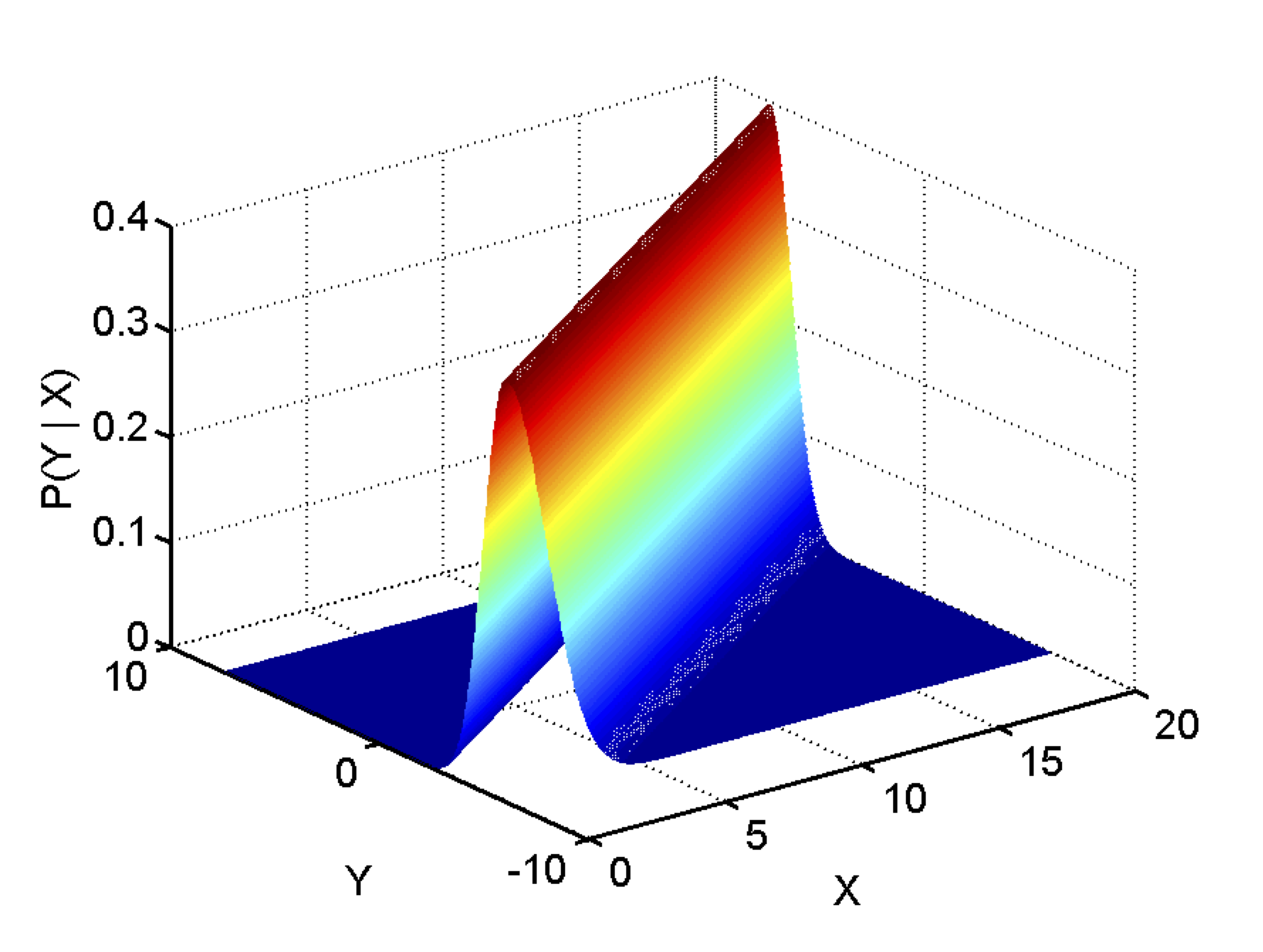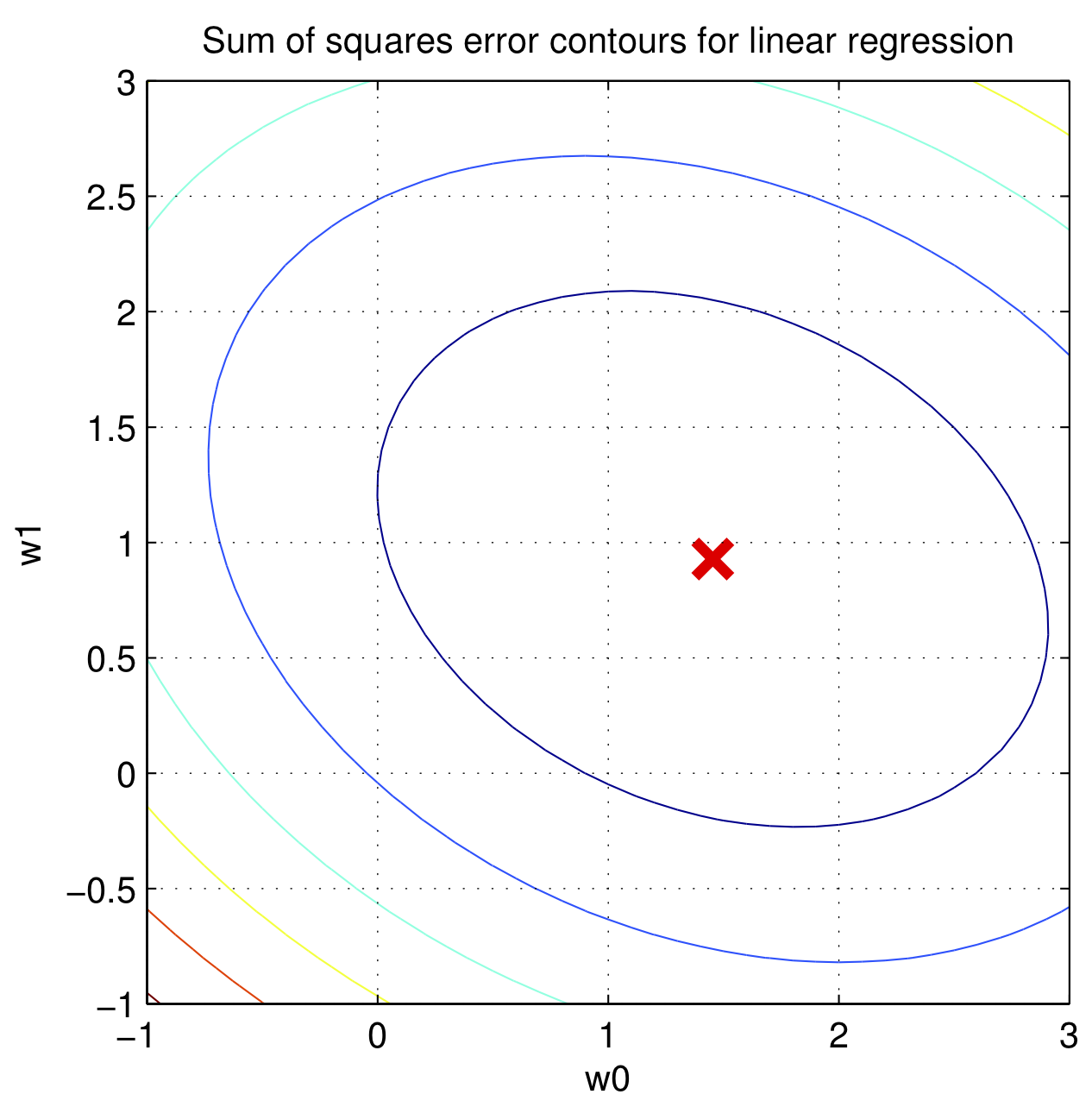$w^TX^Ty$ 和 $y^TXw$ 得到的结果都是 $1\times1$ 的标量，对于标量 $a$，$a^T = a$，因此 $w^TX^Ty = (w^TX^Ty)^T = y^TXw$。

$$\begin{equation} \label{rss} \begin{split} \epsilon^{T}\epsilon & = y^Ty - (Xw)^Ty - y^TXw + (Xw)^TXw \\ & = y^Ty - 2w^TX^Ty + w^TX^TXw \end{split} \end{equation}$$

$$\nabla_{w}w^Ta = \begin{bmatrix} \frac{\partial w^Ta}{\partial w_0}\\ \frac{\partial w^Ta}{\partial w_1}\\ \frac{\partial w^Ta}{\partial w_2} \end{bmatrix} = \begin{bmatrix} a_0\\ a_1\\ a_2 \end{bmatrix} = a$$

$$\begin{equation} \begin{split} w^TAw &= \begin{bmatrix}w_0 & w_1 & w_2\end{bmatrix}\begin{bmatrix} a_{11} & a_{12} & a_{13} \\ a_{12} & a_{22} & a_{23} \\ a_{13} & a_{23} & a_{33} \end{bmatrix}\begin{bmatrix} w_0 \\ w_1 \\ w_2 \end{bmatrix} \\ & = \begin{bmatrix}w_0 & w_1 & w_2\end{bmatrix} \begin{bmatrix} a_{11}w_0 + a_{12}w_1 + a_{13}w_2 \\ a_{12}w_0 + a_{22}w_1 + a_{23}w_2 \\ a_{13}w_0 + a_{23}w_1 + a_{33}w_2 \end{bmatrix} \\ & = \quad w_0(a_{11}w_0 + a_{12}w_1 + a_{13}w_2)\\ & \quad +w_1(a_{12}w_0 + a_{22}w_1 + a_{23}w_2)\\ & \quad +w_2(a_{13}w_0 + a_{23}w_1 + a_{33}w_2) \\ & = a_{11}w_0^2+ a_{22}w_1^2 + a_{33}w_2^2 +2a_{12}w_0w_1+ 2a_{13}w_0w_2+2a_{23}w_1w_2 \end{split} \end{equation}$$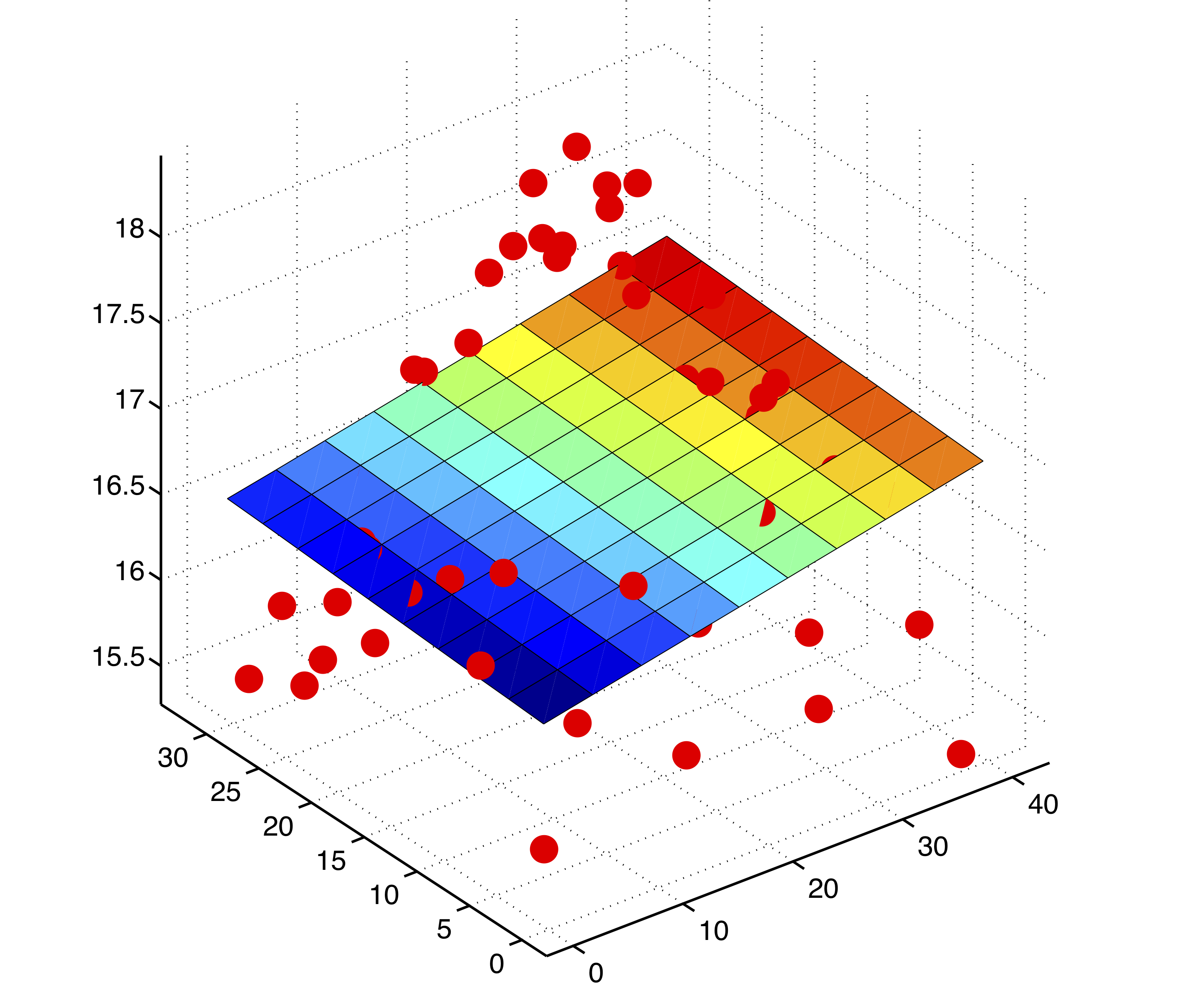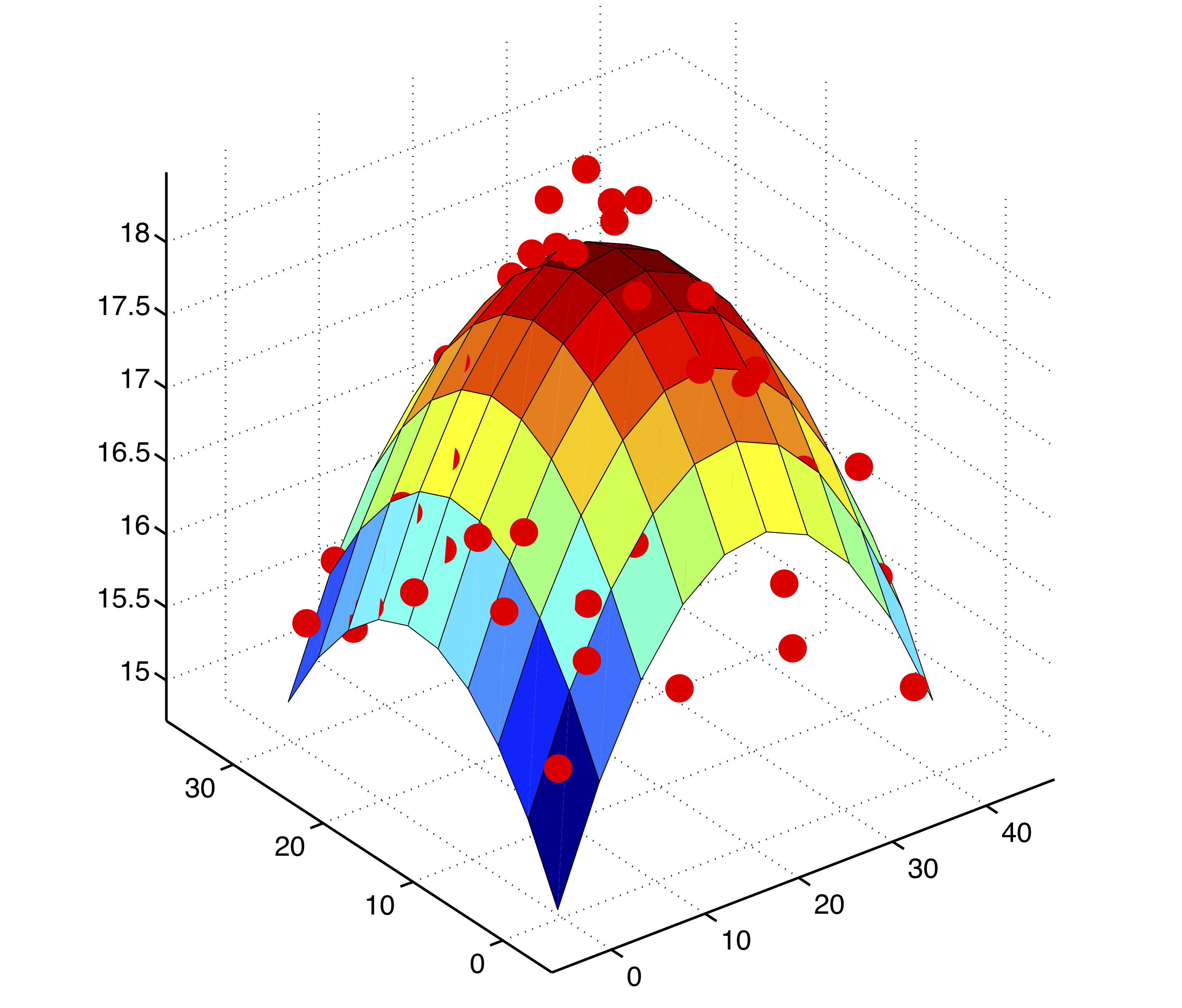## 投影矩阵¶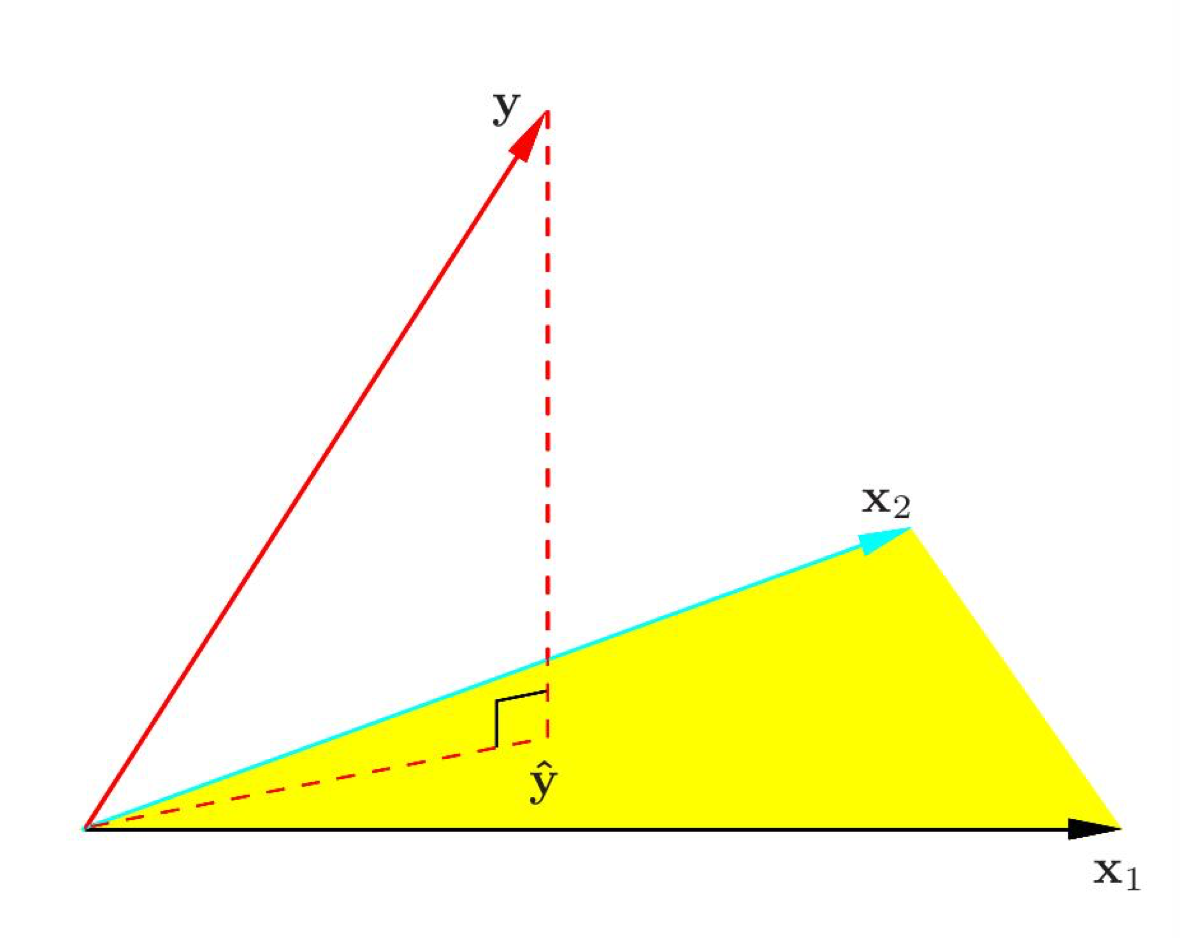$$(y - \hat{y})^Tx_1 = (y - X\hat{w})^Tx_1 = 0\\ (y - \hat{y})^Tx_2 = (y - X\hat{w})^Tx_2 = 0$$

$$\begin{equation} \begin{split} (y-X\hat{w})^TX &= 0\\ X^T(y-X\hat{w}) &= 0\\ X^Ty-X^TX\hat{w} &= 0\\ X^TX\hat{w} &= X^Ty\\ \hat{w} &= (X^TX)^{-1}X^Ty \end{split} \end{equation}$$

## 参考来源¶

Daniel Kahneman 的 "Eureka moment" 参考了维基百科的 Regression toward the mean，第一次听说是 CS109 Joe Blitzstein 讲授的 Bias and Regression。

## 修改与勘误¶

• 修改了最后一段结论。
• $X^TX$ 的逆矩阵存在需要两个条件，1. 线性无关 2. 行数 > 列数，因此修改了文章对 $(X^TX)^{-1}$ 存在的条件和直觉上的理解。